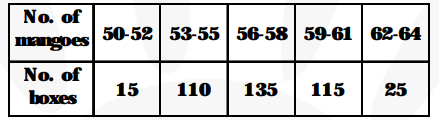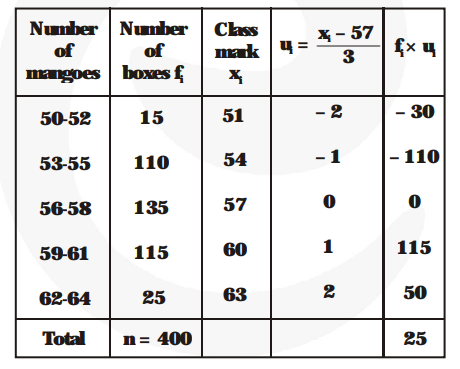# In a retail market, fruit vendors were selling mangoes kept in packing boxes.

Question:

In a retail market, fruit vendors were selling mangoes kept in packing boxes. These boxes contained varying number of mangoes. The following was the distribution of mangoes according to the number of boxes.Find the mean number of mangoes kept in a packing box. Which method of finding the mean did you choose?

Solution:$\mathrm{a}=57, \mathrm{~h}=2, \mathrm{n}=400$ and $\Sigma \mathrm{f}_{\mathrm{i}} \mathrm{u}_{\mathrm{i}}=25$.

By step deviation method,

Mean $=\mathrm{a}+\mathrm{h} \times \frac{\mathbf{1}}{\mathbf{n}} \times \Sigma \mathrm{f}_{\mathrm{i}} \mathrm{u}_{\mathrm{i}}=57+2 \times \frac{\mathbf{1}}{\mathbf{4 0 0}} \times 25=57.19$